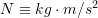### Introduction: Fundamental concepts and principles

#### Basic quantities

A physical quantity is a property that can be quantified by measurement. A physical quantity is measured by comparison with a standard. The unit of measurement is a unique name assigned to a measure of a quantity. For example, meter is a unit of length. The standard for length, corresponding to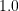unit of meter, is defined by the distance traveled by light in a vacuum during a certain fraction of time unit.

One should distinguish between the concepts of dimension and unit. A dimension is a physical quantity that can be measured (e.g. length, time, temperature, etc.), whereas a unit is a name correlating with the dimension to make it relative.A dimension can be expressed in different units all being related through conversion factors. For example, length is a dimension with meter and foot as two of its units such thatmeter is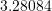feet.

Length, time, and mass are three basis measures or dimensions with the following definitions:

Length. Length is a measure of distance between two points in space. Length is used to measure the relative positions of points in space to a fixed point. Some units of length are meter, inch, foot, yard, etc. The dimension of length is denoted by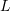.

Time. Time is understood as the succession of events. In physics, time is defined by its measurements, i.e. what a clock (a device for measuring time) reads. Some units of time are second, minute, hour, day, year, century, etc. The dimension of time is denoted by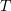.

Mass. Mass measures the quantity (how much) of matter. It also measures the resistance of a body to acceleration (change in velocity) when subjected to net force, or measures the gravitational attraction between two bodies. The units of mass are kilogram, pound, slug, etc. The dimension of mass is denoted by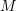.

Other quantities: Generally, the three basic quantities mass, length, and time creates a system of dimensions called the MLT system. There are many other physical quantities that their dimensions are derived based on the above three basic dimensions. For example: area has the dimension of length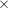length being written as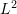. Some others are:

 Physical quantity Dimension Volume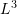Velocity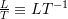Acceleration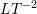Force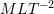Angle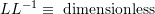Force is a quantity perceived by push or pull exerted by one body onto another. Force is related to the basic quantities through the second Newton’s Law. The concept of force will be fully covered in section #.#.

As a remark, an angle is a physical quantity that has no dimension in the MLT system. Why? The reason emerges by noting the definition and measure of an angle. An angle is defined as the figure formed by two rays, i.e. two half-lines sharing a point and starting from that point. The measure of an angle is the ratio of the length of a circular arc made by the angle to its radius. Since both the length of the arc and the length of the radius have the same dimension, their ratio becomes dimensionless. Common units for measuring angles are Radian and degree.

#### Idealizations

Idealizations or models are simplifications of phenomena so that calculations based on theories and laws can focus on practical matters.

Particle. A particle is a localized object that have mass but its size can be neglected. This means that the size and geometry of an object regarded as a particle does not significantly affect our calculations. For example, any object (e.g. a car, a cat, the earth) can be simplified into a particle when we study their motion from one location to another.

Rigid body: A rigid body is an object that does not experience deformations when being loaded (e.g. pressed or pulled). The distance between two points on/in a rigid body is always the same, whether or not a force is applied to the object. As a result, the shape of a rigid body does not change. In reality, all objects are deformable; however, a rigid-body idealization is suitable in many problem-solving situations in which the deformations are relatively small.

#### International System of Units (SI)

A system of unit is a collection of units of measurements. Two common systems of units are the International System of Units (SI) and the U.S. Customary system of units (FPS). The SI is used through this book. The following table shows the SI units of some quantities often used in mechanics:

Quantity Unit name Unit notation
Length meterTime secondMass kilogram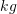Area square meter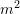Volume cubic meter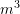Velocity meter per second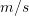Acceleration meter per second squared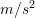Density kilogram per cubic meter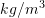Force newton